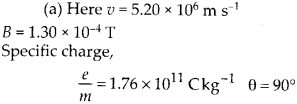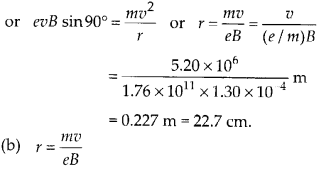Enlightened

# Question 21: NCERT Solutions for 12th Class Physics: Chapter 11-Dual Nature of Radiation and Matter

• 0

Question 21: NCERT Solutions for 12th Class Physics: Chapter 11-Dual Nature of Radiation and Matter

(a) A monoenergetic electron beam with electron speed of 5.20 × 106 m s-1 is subjected to a magnetic field of 1.30 × 10-4 T, normal to the beam velocity. What is the radius of the circle traced by the beam, given e/m for electron equals 1.76 × 1011 C
kg-1.
(b) Is the formula you employ in (a) valid for calculating radius of the path of a 20 MeV electron beam? If not, in what way is it modified?

Share

1. Solution:The normal magnetic field provides necessary centripetal force to the electron beam so that it can follow a circular path. Thus Force on an electron = Centripetal force due to magnetic field on an electronThe formula for radius of circular path is not valid at very high energies because such high energyCheck the complete chapter with solutions.
NCERT Solutions for 12th Class Physics: Chapter 11-Dual Nature of Radiation and Matter

• 0